Chin. Phys. B, 2020, Vol. 29(11): 110503    DOI: 10.1088/1674-1056/ab9ded
 GENERAL Prev   Next

# Nonlinear dynamics in non-volatile locally-active memristor for periodic and chaotic oscillations

Wen-Yu Gu(谷文玉)1, Guang-Yi Wang(王光义)1,†(), Yu-Jiao Dong(董玉姣)1, Jia-Jie Ying(应佳捷)1
Institute of Modern Circuits and Intelligent Information, Hangzhou Dianzi University, Hangzhou 310018, China
 Abstract  Complexity and abundant dynamics may arise in locally-active systems only, in which locally-active elements are essential to amplify infinitesimal fluctuation signals and maintain oscillating. It has been recently found that some memristors may act as locally-active elements under suitable biasing. A number of important engineering applications would benefit from locally-active memristors. The aim of this paper is to show that locally-active memristor-based circuits can generate periodic and chaotic oscillations. To this end, we propose a non-volatile locally-active memristor, which has two asymptotically stable equilibrium points (or two non-volatile memristances) and globally-passive but locally-active characteristic. At an operating point in the locally-active region, a small-signal equivalent circuit is derived for describing the characteristics of the memristor near the operating point. By using the small-signal equivalent circuit, we show that the memristor possesses an edge of chaos in a voltage range, and that the memristor, when connected in series with an inductor, can oscillate about a locally-active operating point in the edge of chaos. And the oscillating frequency and the external inductance are determined by the small-signal admittance Y(iω). Furthermore, if the parasitic capacitor in parallel with the memristor is considered in the periodic oscillating circuit, the circuit generates chaotic oscillations. Keywords:  memristor      chaos local activity      Hopf bifurcation Received:  22 April 2020      Revised:  07 June 2020      Published:  03 November 2020 Fund: the National Natural Science Foundation of China (Grant No. 61771176). Corresponding Authors:  †Corresponding author. E-mail: wanggyi@163.com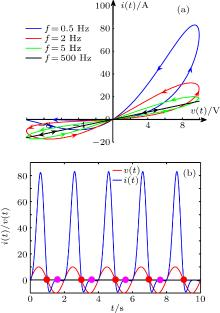Fig. 1.  (a) Pinched hysteresis loops driven by sinusoidal voltage with amplitude A = 10 V at f = 0.5 Hz, 2 Hz, 5 Hz, and 500 Hz, with m = 0 and initial state x(0) = 0.5; (b) time domain waveforms of input voltage (red) and output current (blue) at frequency f = 2 Hz.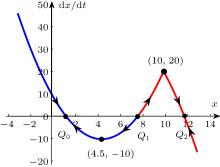Fig. 2.  POP of locally-active memristor.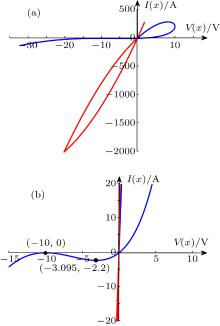Fig. 3.  (a) DC V–I plot of memristor (b) zoomed DC V–I plot near negative slope region.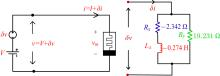Fig. 4.  Small-signal equivalent circuit of memristor at operating point Q(X, V) under exciting voltage δ v.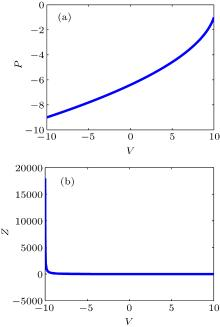Fig. 5.  The pole-zero diagram of the memristor in the voltage range of −10 ≤ V ≤ 10.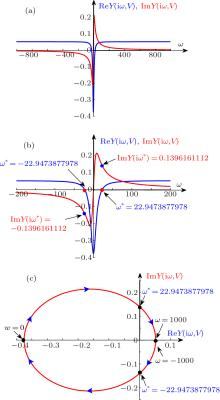Fig. 6.  Frequency response and Nyquist plot of the locally-active memristor at voltage V = −8 V, showing (a) real part Re Y(iω, V) and imaginary Im Y(iω, V) part of frequency response, (b) zoomed Fig. 6(a) in range −200 rad/s ≤ ω ≤ 200 rad/s, and (c) Nyquist plot.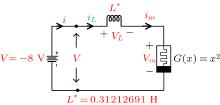Fig. 7.  Oscillating circuit composed of memristor operating in locally-active region.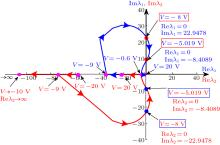Fig. 8.  Trajectories of eigenvalues in range of −20 V ≤ V ≤ 20 V.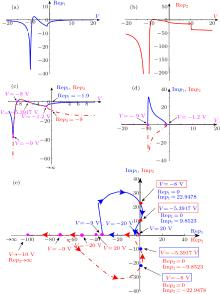Fig. 9.  Pole-zero diagrams of Yc(s, V) for (a) real part of pole p1, (b) real part of pole p2, (c) zoomed real part of poles p1 and p2 in the range of −10 V ≤ V ≤ 10 V, (d) imaginary part of poles p1 and p2, (e) the relationship between the real and imaginary parts of poles p1 and p2, in which the arrowheads indicate the movement direction of the pole as the voltage V increases.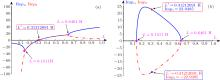Fig. 10.  Pole diagrams of admittance Yc(s, V) with external inductance L in a range of 0 < L < 1 H, showing (a) real part of the poles, p1 and p2, and (b) imaginary part of the poles.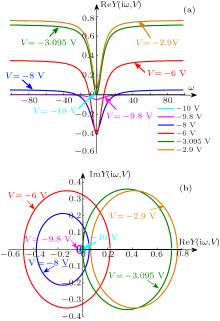Fig. 11.  Frequency response of admittance function Y(iω, V) with respect to ω, showing (a) real part curve of frequency response of memristor in a range of −100 rad/s ≤ ω ≤ 100 rad/s, and (b) Nyquist plot of memristor frequency response in a range of −1000 rad/s ≤ ω ≤ 1000 rad/s.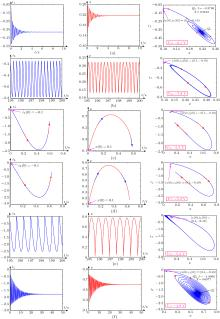Fig. 12.  Transient waveforms of state variable x and current iL with different DC voltages, where initial states are (x(0), iL(0)) = (0.1, −0.1) (a) DC voltage V = −8.1 V, and transient waveforms converge to stable equilibrium point Q0; (b) DC voltage V = −7.8 V, and the system oscillates to form a limit cycle; (c) DC voltage V = −5.5 V, and the system is in unstable state, diverging from initial state; (d) DC voltage V = −5.3 V, and the system is in unstable state, diverging from initial state; (e) DC voltage V = −5.2 V, and the system oscillates to form a limit cycle; (f) DC voltage V = −4.9 V, transient waveforms converge to stable equilibrium point ${Q}_{0}^{^{\prime} }$.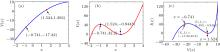Fig. 13.  Plots of the memristor determined by Eqs. (38) and (39): (a) $V=\mathop{v}\limits^\unicode{x2323}(X)$, (b) $I=\mathop{i}\limits^\unicode{x2323}(X)$, and (c) DC V–I plot.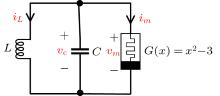Fig. 14.  Chaotic oscillator circuit based on locally-active memristor.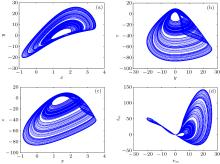Fig. 15.  Phase diagrams of this memristor-based chaotic oscillating circuit (a) x–y plane, (b) y–z plane, (c) x–z plane, and (d) chaotic pinched hysteresis loops of the memristor.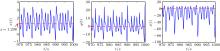Fig. 16.  Time domain waveform of memristor state variable x, capacitor voltage vC, and inductor current iL.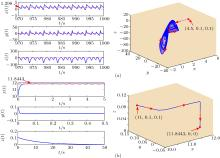Fig. 17.  (a) Time domain waveforms and x–y–z phase diagrams with circuit parameters fixed at (a) (x0,y0, z0) = (4.5, 0.1, 0.1); (b) (x0, y0, z0) = (11, 0.1, 0.1), but the initial values changed.Table 1.  Nonlinear dynamic characteristics of different initial conditions.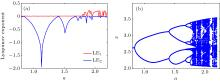Fig. 18.  (a) Lyapunov exponent (LE) spectrum and (b) bifurcation diagram varying with parameter a.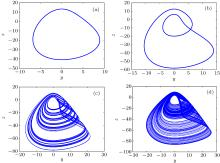Fig. 19.  Phase diagram changing with parameter a on the x–y plane: (a) a = 0.8, (b) a = 1.2, (c) a = 1.793, and (d) a = 2.13.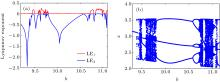Fig. 20.  (a) Lyapunov exponent spectrum and (b) bifurcation diagram changing with parameter b.